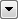You are here: Rasters > RasterExpressionEvaluator

# RasterExpressionEvaluator

Evaluates expressions on each cell in a raster, such as algebraic operations or conditional statements.

## Input Ports

Features are input through ports A and B. The cardinality of the input is required to be one of the following cases:

• one or more As, no Bs
• one A, one or more Bs

Note that the B port is only visible if Two Rasters is specified in the Mode parameter.

When both A and B features are provided, the single A input will be paired with each B input.

Note the following restrictions on input features:

• Input features must have raster geometry.
• All paired rasters must have the same number of rows and columns.
• Either all bands used in the same expression must have the same nodata value, or all bands used in the same expression must have no nodata value.
• No band may have a palette.

## Usage Notes

Note that when converting between different data types, a Bounded Cast is used. As a result, when a calculated value does not fit in the specified destination interpretation, the corresponding destination value will either be set to the minimum or maximum value possible in the destination data type.

## Constructing Expressions

The Arithmetic Editor - Raster Expression is available via the Expression ellipsis (...) button to assist in the construction of expressions.

Expression Syntax

Means...

|| Or
&& And
== Equal
!= Not equal
< Less than
> Greater than
A[x] Band x of raster feature A
B[x] Band x of raster feature B

Bands start counting at zero (so a three-band raster is A, A, and A).

A statement can be as simple as these:

 255 Set the value of the current cell to be 255. A+1 Set the value of the current cell to be Raster A, band zero, plus 1.

"if" statements allow a value to be set depending on conditions, in the form if (conditions, pass value, fail value):

 if (A < 255, 200, 255) If Raster A, band zero is less than 255, then set the current cell to 200, else leave it as 255. if (A<50 || A<50 || A<50, 0, 255) If Raster A, band zero is less than 50 OR band one is less than 50 OR band two is less than 50, then set the current cell to 0, else set it to 255.

Bands in two raster features can be referenced in the statement:

 if (A!=B && A<255, 0, A) If Raster A band zero is not equal to Raster B band zero, AND Raster A band zero is less than 255, then set the current cell to 0, else set it to the value of Raster A band zero.

Standard maths operators are permitted:

 if (A - B == 255, 0, A/5) If Raster A band zero minus Raster B band zero equals 255, then set the current cell to 0, else set it to the value of Raster A band zero divided by five.

Attributes can be referenced using @Value and either A: or B: to refer to the attribute name:

 A*@Value(A:myattr) Multiply Raster A band zero by the attribute myattr on raster A.

Examples

Expression

Result

255-A Inverts an 8-bit band. For a 24-bit RGB image, repeat for A and A to invert the full image, repeat for A too if it is a 32-bit RGBA image
if ((A+A+A)==0, 255, A) Convert black values into white in a 24-bit RGB image. Repeat for A and A to invert the full image
A*1.5 Increases the brightness of the red band in an RGB image. Repeat for A and A to brighten the full image
@sqrt((A*A) + (B*B)) Combines two raster cells together. An alternative would be to average the values: (A+B)/2)
if(A==0,0,A/@Value(A:_height+1)) Classify raster DEM cells into groups where group size is defined by the _height attribute

## Editing Transformer Parameters

Using a set of menu options, transformer parameters can be assigned by referencing other elements in the workspace. More advanced functions, such as an advanced editor and an arithmetic editor, are also available in some transformers. To access a menu of these options, clickbeside the applicable parameter. For more information, see Transformer Parameter Menu Options.

## FME Licensing Level

FME Professional edition and above